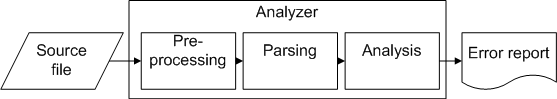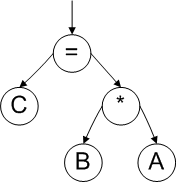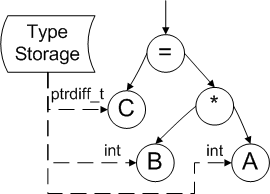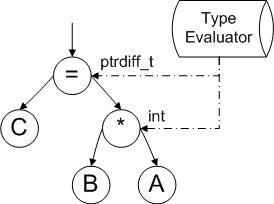﻿ Static code analysis for verification of the 64-bit applications# Static code analysis for verification of the 64-bit applications

The coming of 64-bit processors to the PC market causes a problem which the developers have to solve: the old 32-bit applications should be ported to the new platform. After such code migration an application may behave incorrectly. The article is elucidating question of development and appliance of static code analyzer for checking out of the correctness of such application. Some problems emerging in applications after recompiling in 64-bit systems are considered in this article as well as the rules according to which the code check up is performed.

This article contains various examples of 64-bit errors. However, we have learnt much more examples and types of errors since we started writing the article and they were not included into it. Please see the article "A Collection of Examples of 64-bit Errors in Real Programs" that covers defects in 64-bit programs we know of most thoroughly. We also recommend you to study the course "Lessons on development of 64-bit C/C++ applications" where we describe the methodology of creating correct 64-bit code and searching for all types of defects using the Viva64 code analyzer.

## 1. Introduction

Mass production of the 64-bit processors and the fact that they are widely spread led the developers to the necessity to develop 64-bit versions of their programs. The applications must be recompiled to support 64-bit architectures exactly for users to get real advantages of the new processors. Theoretically, this process must not contain any problems. But in practice after the recompiling an application often does not function in the way it is supposed to do. This may occur in different situations: from data file failure up to help system break down. The cause of such behavior is the alteration of the base type data size in 64-bit processors, to be more exact, in the alteration of type size ratio. That's why the main problems of code migration appear in applications which were developed using programming languages like C or C++. In languages with strictly structuralized type system (for example .NET Framework languages) as a rule there are no such problems.

So, what's the problem with exactly these languages? The matter is that even all the high-level constructions and C++ libraries are finally realized with the use of the low-level data types, such as a pointer, a machine word, etc. When the architecture is changed and these data types are changed, too, the behavior of the program may also change.

In order to be sure that the program is correct with the new platform it is necessary to check the whole code manually and to make sure that it is correct. However, it is impossible to perform the whole real commercial application check-up because of its huge size.

## 2. The example of problems arising when code is ported to 64-bit platforms

Here are some examples illustrating the appearance of some new errors in an application after the code migration to a 64-bit platform. Other examples may be found in different articles [1, 2].

When the amount of memory necessary for the array was defined constant size of type was used. With the 64-bit system this size was changed, but the code remained the same:

``````size_t ArraySize = N * 4;
intptr_t *Array = (intptr_t *)malloc(ArraySize);``````

Some function returned the value of -1 size_t type if there was an error. The checking of the result was written in the following way:

``````size_t result = func();
if (result == 0xffffffffu) {
// error
}``````

For the 64-bit system the value of -1 for this type is different from 0xffffffff and the check up does not work.

The pointer arithmetic is a permanent source of problems. But in the case of 64-bit applications some new problems are added to the already existing ones. Let's consider the example:

``````unsigned a16, b16, c16;
char *pointer;
...
pointer += a16 * b16 * c16;``````

As we can see, the pointer is never able to get an increment more than 4 gigabytes and this, though, is not diagnosed by modern compilers as a warning, and in the future would lead to disability of programs to work. There exist many more examples of potentially dangerous code.

All these and many other errors were discovered in real applications while migration to the 64-bit platform.

## 3. The review of the existing solutions

There exist different approaches to the securing of the code applications correctness. Let's enumerate the most widely spread ones: unit test checking, dynamic code analysis (performed when an application is working), static code analysis (analysis of source code). No one can claim that one of the variants of testing is better than the others, but all these approaches support different aspects of application quality.

Unit tests are meant for quick checking of small sections of a code, for instance, of single functions and classes . Their peculiarity is that these tests are performed quickly and allow being started often. And this causes two nuances of using this technology. The first one is that these tests must be written. Secondly, testing of large amounts of memory (for example, more than two gigabytes) takes much time, so it is not expedient because the unit tests must work fast.

Dynamic code analyzers (the best representative of which is Compuware Bounds Checker) are meant to find errors in an application while the latter is running a program. This principle of work determines the main disadvantage of the dynamic analyzer. To make sure the program is correct it is necessary to accomplish all the possible code branches. For a real program this might be difficult. But this does not mean that the dynamic code analyzer is useless. This analysis allows to discover the errors which depends upon the actions of the user and cannot be defined through the application code.

Static code analyzers (for instance Gimpel Software PC-lint and Parasoft C++test) are meant for complex securing of the code quality and contain several hundreds of analyzed rules . They also contain some rules which analyze the correctness of 64-bit applications. However, they are code analyzers of general purpose, so their use of securing the 64-bit application quality is not always appropriate. This can be explained by the fact that they are not meant for this purpose. Another serious disadvantage is their directivity to the data model which is used in Unix-systems (LP64),while the data model used in Windows-systems (LLP64) is quite different. That's why the use of static analyzers for checking of 64-bit Windows applications can be possible only after unobvious additional setting.

The presence of special diagnostic system for potentially incorrect code (for instance key /Wp64 in Microsoft Visual C++ compiler) can be considered as some additional level of code check. However this key allows to track only the most incorrect constructions, while it leaves out many other dangerous operations.

There arises a question "Is it really necessary to check the code while migrating to 64-bit systems if there are only few such errors in the application?" We believe that this checking is necessary at least because large companies (such as IBM and Hewlett-Packard) have laid out some articles  devoted to errors which appear when the code is being ported in their sites.

## 4. The Rules of the Code Correctness Analysis

We have formulated 10 rules of search of dangerous from the point of view of code migrating to 64-bit system C++ language constructions.

In the rules we use a specially introduced memsize type. Here we mean any simple integer type capable of storing a pointer inside and able to change its size when the digit capacity of a platform changes from 32 to 64 bit. The examples of memsize types are size_t, ptrdiff_t, all pointers, intptr_t, INT_PTR, DWORD_PTR.

Now let's list the rules themselves and give some examples of their application.

### RULE 1

Constructions of implicit and explicit integer type of 32 bits converted to memsize types should be considered dangerous:

``````unsigned a;
size_t b = a;
array[a] = 1;``````

The exceptions are:

1) The converted 32-bit integer type is a result of an expression in which less than 32 bits are required to represent the value of an expression:

``````unsigned short a;
unsigned char b;
size_t c = a * b;``````

At the same time the expression must not consist of only numerical literals:

``size_t a = 100 * 100 * 100;``

2) The converted 32-bit type is represented by a numeric literal:

``````size_t a = 1;
size_t b = 'G';``````

### RULE 2

Constructions of implicit and explicit conversion of memsize types to integer types of 32-bit size should be considered dangerous:

``````size_t a;
unsigned b = a;``````

An exception: the converted size_t is the result of sizeof() operator accomplishment:

``int a = sizeof(float);``

### RULE 3

We should also consider to be dangerous a virtual function which meets the following conditions:

a) The function is declared in the base class and in the derived class.

b) Function argument types does not coincide but they are equivalent to each other with a 32-bit system (for example: unsigned, size_t) and are not equivalent with 64-bit one.

``````class Base {
virtual void foo(size_t);
};
class Derive : public Base {
virtual void foo(unsigned);
};``````

### RULE 4

The call of overloaded functions with the argument of memsize type. And besides, the functions must be overloaded for the whole 32-bit and 64-bit data types:

``````void WriteValue(__int32);
void WriteValue(__int64);
...
ptrdiff_t value;
WriteValue(value);``````

### RULE 5

The explicit conversion of one type of pointer to another should be consider dangerous if one of them refers to 32/64 bit type and the other refers to the memsize type:

``````int *array;
size_t *sizetPtr = (size_t *)(array);``````

### RULE 6

Explicit and implicit conversion of memsize type to double and vice versa should be considered dangerous:

``````size_t a;
double b = a;``````

### RULE 7

The transition of memsize type to a function with variable number of arguments should be considered dangerous:

``````size_t a;
printf("%u", a);``````

### RULE 8

The use of series of magic constants (4, 32, 0x7fffffff, 0x80000000, 0xffffffff) should be considered as dangerous:

``````size_t values[ARRAY_SIZE];
memset(values, ARRAY_SIZE * 4, 0);``````

### RULE 9

The presence of memsize types members in unions should be considered as dangerous:

``````union PtrNumUnion {
char *m_p;
unsigned m_n;
} u;
...
u.m_p = str;
u.m_n += delta;``````

### RULE 10

Generation and processing of exceptions with use of memsize type should be considered dangerous:

``````char *p1, *p2;
try {
throw (p1 - p2);
}
catch (int) {
...
}``````

It is necessary to note the fact that rule 1 covers not only type conversion while it is being assigned, but also when a function is called, an array is indexated and with pointer arithmetic. These rules (the first as well as the others) describe a large amount of errors, larger than the given examples. In other words, the given examples only illustrate some particular situations when these rules are applied.

The represented rules are embodied in static code analyzer Viva64. The principle of its functioning is covered in the following part.

## 5. Analyzer architecture

The work of analyzer consists of several stages, some of which are typical for common C++ compilers (picture 1).Picture 1. Analyzer architecture.

At the input of the analyzer we have a file with the source code, and as a result of its work a report about potential code errors (with line numbers attached) is generated. The stages of the analyzer's work are the following: preprocessing, parsing and analysis itself.

At the preprocessing stage the files introduced by means of #include directive are inserted, and also the parameters of conditional compiling (#ifdef/#endif) are processed.

After the parsing of a file we get an abstract syntax tree with the information necessary for the future analysis is constructed. Let's take up a simple example:

``````int A, B;
ptrdiff_t C;
C = B * A;``````

There is a potential problem concerned with different data types in this code. Variable C can never possess the value less or more than 2 gigabytes and such situation may be incorrect. The analyzer must report that there is a potentially incorrect construction in the line "C = B * A". There are several variants of correction for this code. If variables B and a cannot possess the value less or more than 2 gigabytes in terms of the value, but the variable C can do it, so the expression should be written in the following way:

``C =  (ptrdiff_t)(B) * (ptrdiff_t)(A);``

But if the variables A and B with a 64-bit system can possess large values, so we should replace them with ptrdiff_t type:

``````ptrdiff_t A;
ptrdiff _t B;
ptrdiff _t C;
C = B * A;``````

Let's see how all this can be performed at the parsing stage.

First, an abstract syntax tree is constructed for the code (picture 2).Picture 2. Abstract syntax tree.

Then, at the parsing stage it is necessary to determine the types of variables, which participate in the evaluation of the expression. For this purpose some auxiliary information is used. This information was received when the tree was being constructed (type storage module). We can see this on the picture 3.Picture 3. Type Information storage.

After the determination of types of all the variables participating in the expression it is necessary to calculate the resulting types of subexpressions. In the given example it is necessary to define the type of result of the intermediate expression "B * A". This can be done by means of the type evaluation module, as it is shown on the picture 4.Picture 4. Expression type evaluation.

Then the correction of the resulting type expression evaluation is performed (operation "=" in the given example) and in the case of type conflict the construction is marked as potentially dangerous. There is such a conflict in the given example, because the variable C possesses the size of 64 bits (with the 64-bt system) and the result of the expression "B * A" possesses the size of 32 bits.

The analysis of other rules is performed in the similar way because almost all of them are related to the correction of the types of one or another parameter.

## 6. Results

Most methods of code analysis described in this article are embodied in the commercial static code analyzer Viva64. The use of this analyzer with real projects has proved the expediency of the code checking while developing 64-bit applications - real code errors could be discovered much quicker by means of this analyzer, than if you just use common examination of the source codes.

## References

• J. P. Mueller. "24 Considerations for Moving Your Application to a 64-bit Platform", DevX.com, June 30, 2006.
• Hewlett-Packard, "Transitioning C and C++ programs to the 64-bit data model".
• S. Sokolov, "Bulletproofing C++ Code", Dr. Dobb's Journal, January 09, 2007.
• S. Meyers, M. Klaus, "A First Look at C++ Program Analyzer", Dr. Dobb's Journal, Feb. Issue, 1997.##### Use PVS-Studio to search for bugs in C, C++, C# and Java

We offer you to check your project code with PVS-Studio. Just one bug found in the project will show you the benefits of the static code analysis methodology better than a dozen of the articles.

411
14 100

### Do you make errors in the code?